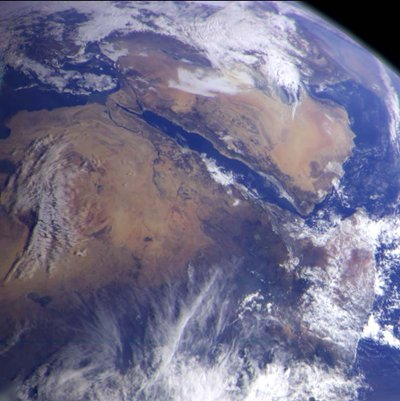Earth is the third smallest planet in the Solar System and is the third planet from the sun. It is the densest planet and is the largest of the four terrestrial planets (Mercury, Venus, Earth and the dwarf planet Ceres).

Earth’s mass is 5.515g/cm. Its volume is 1.08321 × 1012 km. It has a diameter of 8,000 miles or 13,000 km. The planet which closely resembles the Earth in its mass, density and volume is Venus. Mercury has almost the same density as earth but is relatively smaller in mass.

Earth is not a perfect circle. It’s actually a spheroid. The reason for its circular shape is because of its gravity. Gravity is the force which pulls objects towards its center. The earth is also not perfectly round as it has a bulge in its center (the equator). This is due to the earth’s rotation.

The Earth’s mass was calculated through the efforts of Erasthothenes, Cavendish, Galileo and Newton. As you can imagine there is no measuring tape long enough to go around the diameter of the earth so mathematics was used in order to determine the size of the earth. Through the combined efforts of these astronomers and mathematicians, overtime a formula was created in order to find the mass of the earth.

This is the formula to find the gravitational pull of the earth: F=GmM/r2. F is the gravitational force. G is a constant of proportionality. M is the two objects exerting the force and r is the distance between the two objects. Take note that F= Ma. M is mass and A is its acceleration. Once you figure out the force of gravity and divide it by the acceleration due to gravity you will get the mass of the earth, which is 5.515 g/cm.

Scenes of Earth from Space